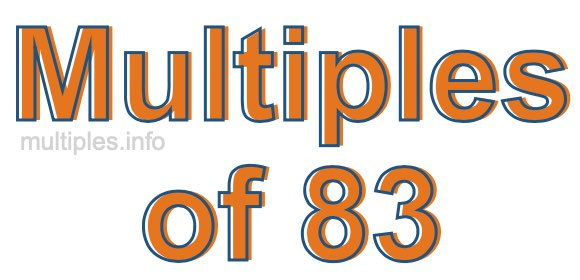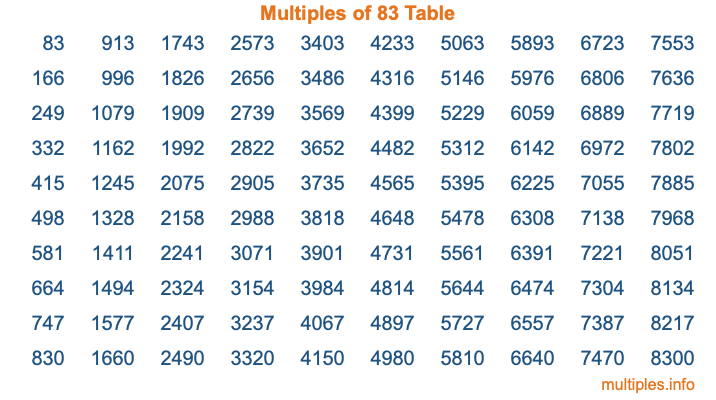Multiples of 83Welcome to the Multiples of 83 page. Here we will first teach you everything you will ever need to know about the multiples of 83, and then give you a study guide summary of everything we taught you to make sure you remember it all. Use this page to look up facts and learn information about the multiples of 83. This page will make you a multiples of eighty-three expert!

Definition of Multiples of 83
Multiples of 83 are all the numbers that when divided by 83 equal an integer. Each of the multiples of 83 are called a multiple. A multiple of 83 is created by multiplying 83 by an integer.

Therefore, to create a list of multiples of 83, you start with 1 multiplied by 83, then 2 multiplied by 83, then 3 multiplied by 83, and so on for as long as you want. Thus, the list of the first five multiples of 83 is 83, 166, 249, 332, and 415. To see a larger list of multiples of 83, see the printable image of Multiples of 83 further down on this page. We also have a category where you can choose any nth multiple of 83.

Multiples of 83 Checker
The Multiples of 83 Checker below checks to see if any number of your choice is a multiple of 83. In other words, it checks to see if there is any number (integer) that when multiplied by 83 will equal your number. To do that, we divide your number by 83. If the the quotient is an integer, then your number is a multiple of 83.

Is  a multiple of 83?

Least Common Multiple of 83 and ...
A Least Common Multiple (LCM) is the lowest multiple that two or more numbers have in common. This is also called the smallest common multiple or lowest common multiple and is useful to know when you are adding our subtracting fractions. Enter one or more numbers below (83 is already entered) to find the LCM.

Check out our LCM Calculator if you need more details about the Least Common Multiple or if you need the LCM for different numbers for adding and subtraction fractions.

nth Multiple of 83
As we stated above, 83 is the first multiple of 83, 166 is the second multiple of 83, 249 is the third multiple of 83, and so on. Enter a number below to find the nth multiple of 83.

th multiple of 83

Multiples of 83 vs Factors of 83
83 is a multiple of 83 and a factor of 83, but that is where the similarities end. All postive multiples of 83 are 83 or greater than 83. All positive factors of 83 are 83 or less than 83.

Below is the beginning list of multiples of 83 and the factors of 83 so you can compare:

Multiples of 83: 83, 166, 249, 332, 415, etc.

Factors of 83: 1, 83

As you can see, the multiples of 83 are all the numbers that you can divide by 83 to get a whole number. The factors of 83, on the other hand, are all the whole numbers that you can multiply by another whole number to get 83.

It's also interesting to note that if a number (x) is a factor of 83, then 83 will also be a multiple of that number (x).

Multiples of 83 vs Divisors of 83
The divisors of 83 are all the integers that 83 can be divided by evenly. Below is a list of the divisors of 83.

Divisors of 83: 1, 83

The interesting thing to note here is that if you take any multiple of 83 and divide it by a divisor of 83, you will see that the quotient is an integer.

Multiples of 83 Table
Below is an image of the first 100 multiples of 83 in a table. The table is in chronological order, column by column. The first column has the first ten multiples of 83, the second column has the next ten multiples of 83, and so on.The Multiples of 83 Table is also referred to as the 83 Times Table or Times Table of 83. You are welcome to print out our table for your studies.

Negative Multiples of 83
Although not often discussed or needed in math, it is worth mentioning that you can make a list of negative multiples of 83 by multiplying 83 by -1, then by -2, then by -3, and so on, to get the following list of negative multiples of 83:

-83, -166, -249, -332, -415, etc.

Multiples of 83 Summary
Below is a summary of important Multiples of 83 facts that we have discussed on this page. To retain the knowledge on this page, we recommend that you read through the summary and explain to yourself or a study partner why they hold true.

There are an infinite number of multiples of 83.

A multiple of 83 divided by 83 will equal a whole number.

83 divided by a factor of 83 equals a divisor of 83.

The nth multiple of 83 is n times 83.

The largest factor of 83 is equal to the first positive multiple of 83.

83 is a multiple of every factor of 83.

83 is a multiple of 83.

A multiple of 83 divided by a divisor of 83 equals an integer.

83 divided by a divisor of 83 equals a factor of 83.

Any integer times 83 will equal a multiple of 83.

Multiples of a Number
Here you can get the multiples of another number, all with the same attention to detail as we did for multiples of 83 on this page.

Multiples of
Multiples of 84
Did you find our page about multiples of eighty-three educational? Do you want more knowledge? Check out the multiples of the next number on our list!